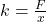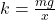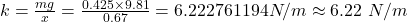## A spring is hung vertically with a 425g mass attached to it. The mass is at rest. If the mass causes the spring to stretch 0.67 m, what is t

Question

A spring is hung vertically with a 425g mass attached to it. The mass is at rest. If the mass causes the spring to stretch 0.67 m, what is the spring constant?

in progress 0
2 months 2021-08-04T02:12:52+00:00 1 Answers 3 views 0

6.22 N/m

Explanation:

From Hooke’s law we deduce that F=kx where F is the applied force and k is the spring constant while x is the extension or compression of the spring. Making k the subject of the above formula thenWe also know that the force F is equal to mg where m is the mass of an object and g is acceleration due to gravity hence substituting F with mg we get thatSubstituting m with 425 g which is equivalent to 0.425 kg and g with 9.81 then 0.67 for x we get thatTherefore, the spring constant is approximately 6.22 N/m